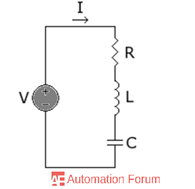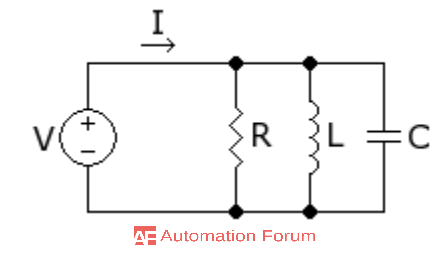# What is RLC circuit and its applications

What is an RLC circuit

The circuit which consists of resistor-capacitor and inductor is an RLC circuit it may be connected in series or in parallel. Thus it forms a harmonic oscillator for current and it will resonate in a similar way as an LC circuit. it could be also called as a second-order circuit as any voltage or current can be described by a second-order differential equation for the analysis of the circuit. RLC circuits are used to tune the frequencies of AM/FM radios it can be used as a bandpass and bandstop filter. Because of the presence of resistor the peak resonant frequency is reduced

The most important property of this circuit is its ability to resonate at a specific frequency

ɯ0 - 2πf0

f0= the resonance frequency ɯ0= angular frequency

Resonance will occur because its energy is stored in two different ways, when the capacitor is charged it stores energy in the form of electric field and in the form of a magnetic field as the current flows through the inductor the transferring of energy from one to the other can be possible in this circuit and it could be oscillatory.

Series RLC circuit

In the series RLC circuit the circuit is constructed by the combination of capacitor, inductor, and resistor and they are connected in series. The three components in this circuit are in series with the voltage source, the RLC series circuit can be used as a bandpass filter.

With the help of the given parameters, we could find the charge Q by using Kirchhoff’s voltage law

VR + VL + VC = V

In series RLC circuit the larger reactance determines the net reactance of the circuit and the current is maximum at resonance.Impedance of series RLC circuit

RLC circuit in series has inductance and capacitance XL and XC, has the opposite effect for the circuit phase angle and thus the total reactance is less than the individual reactance

If XL > XC the circuit is inductive

If XC > XL the circuit is capacitive

Then the total reactance is (XL - XC)

The total impedance of RLC circuit in series is θ= tan-1(Xtot/ R)

Xtot is total reactance

Voltage in series combination of L and C

In a series RLC circuit, the capacitor voltage and the inductor voltage are always 180 degrees out of phase with each other and because of that the VC and VL subtract from each other. If the voltage across the L and C are combined it will always be less than the larger individual voltage.

Series resonance

Series resonance is a condition in a series RLC circuit in which the magnitude of capacitive and inductive reactance are always equal and the result is purely resistive impedance.

Parallel RLC circuit

The parallel RLC is the opposite of the series the applied voltage remains the same in the components and the supplied current gets divided the total current from the supply is not equal to the sum of the flow of the current in individual component but the vector sum is equal as the current flow of the resistor inductor and capacitor are not in the same phase it can’t be added arithmetically.

• Its impedance is infinity at resonance
• In the case of the band-pass filter, the critical frequency is the frequency which is above and below the resonance and the circuit response is 70% of the maximum responseParallel resonant circuit

The parallel resonant circuit will store energy in the magnetic field of the coil and in the capacitors’ electric field, and the energy is transferred back and forth between the coil and capacitor. The impedance is maximum at the resonant frequency for the parallel resonant circuit and the total current will be minimum.

What are the applications of the resonant RLC circuit

• It could be used for the tuning process of an oscillator circuit, radio receiver and television sets
• Series RLC circuit does the signal processing and communication system
• Resonant series LC circuit does the voltage magnification

What are the difference between series and parallel RLC circuit

• Resistor, capacitor, and inductor are connected in series for series RLC, resistor inductor and capacitor are connected in parallel for parallel RLC
• current is the same in each element in the series but it is different in each element for parallel
• Different voltage for each element in series
• voltage across is the same for each element in parallel
• The circuit has minimum impedance when XL = XC at resonance in series the circuit has maximum impedance when XL = XC at resonance in parallel